# 6. In Problem 1, show that θ2 is a consistent estimator for θ. Deduce that Y(n) is a consistent e...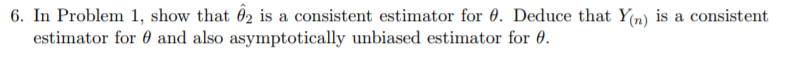Question 1: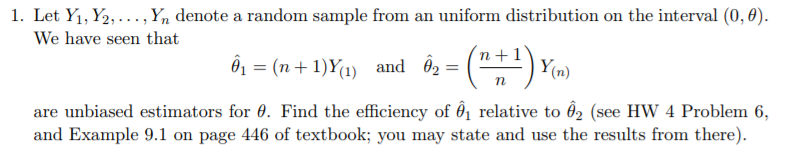6. In Problem 1, show that θ2 is a consistent estimator for θ. Deduce that Y(n) is a consistent estimator for θ and also asyınpt○tically unbiased estimator for θ.
1. Let Yi, ½, . . . ,y, denote a random sample from an uniform distribution on the interval (0,0). We have seen that (1) and 62 Ym are unbiased estimators for 0. Find the efficiency of 6 relative to 62 (see HW 4 Problem 6, and Example 9.1 on page 446 of textbook; you may state and use the results from there)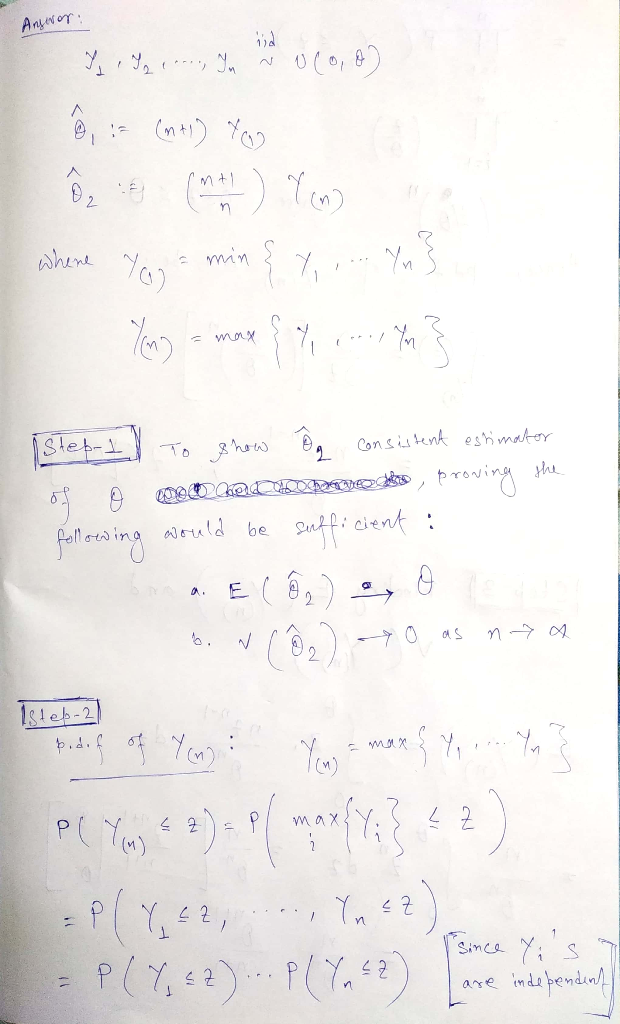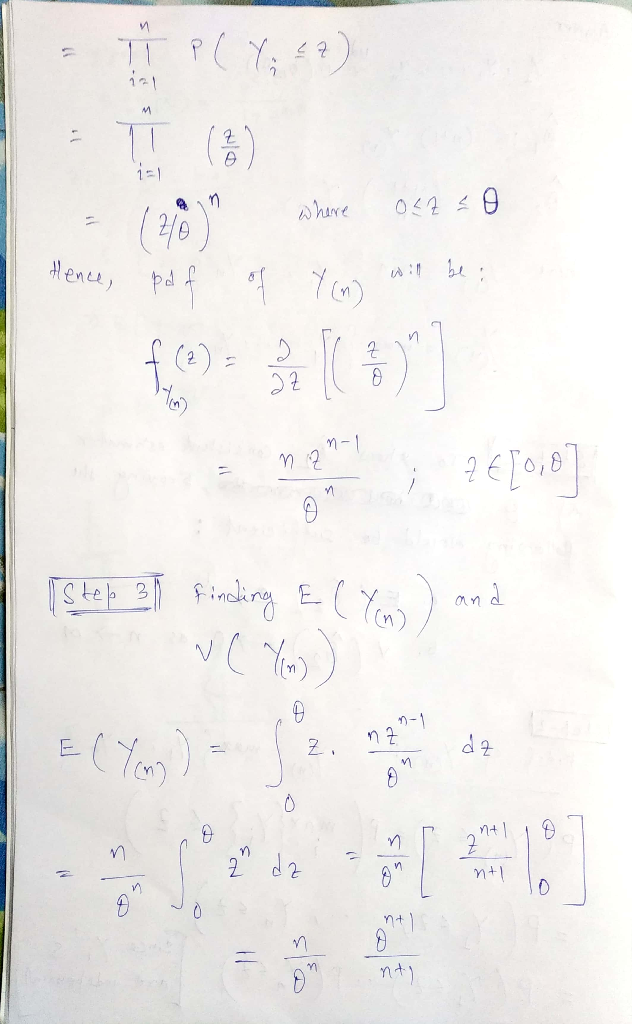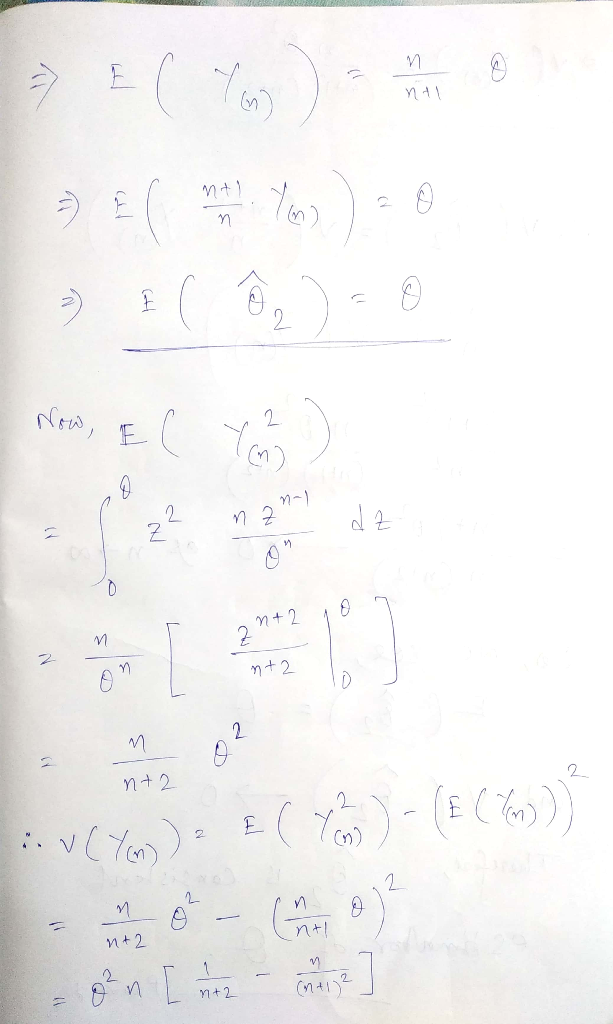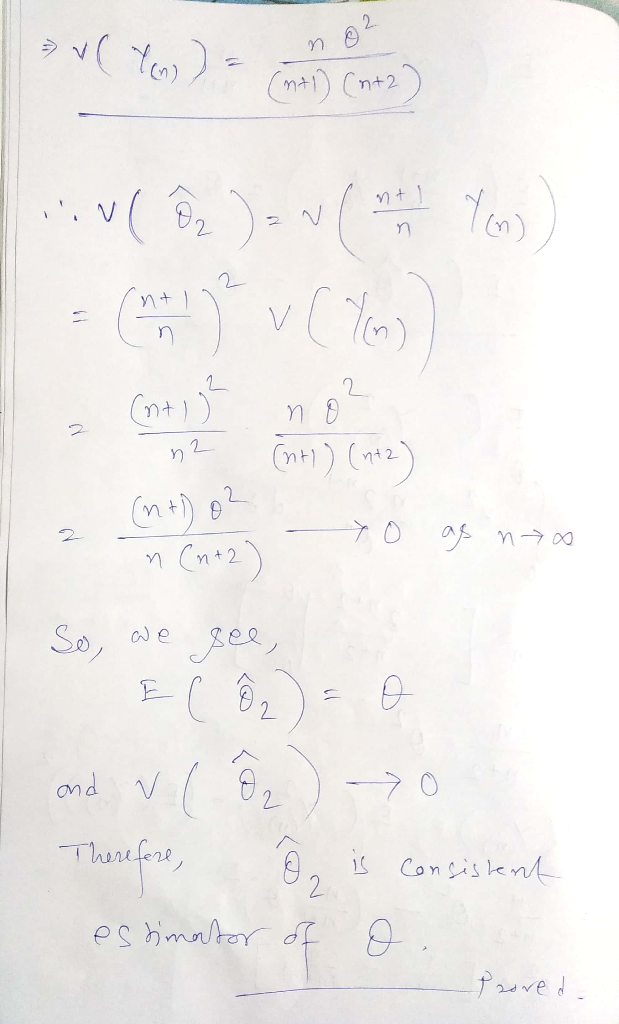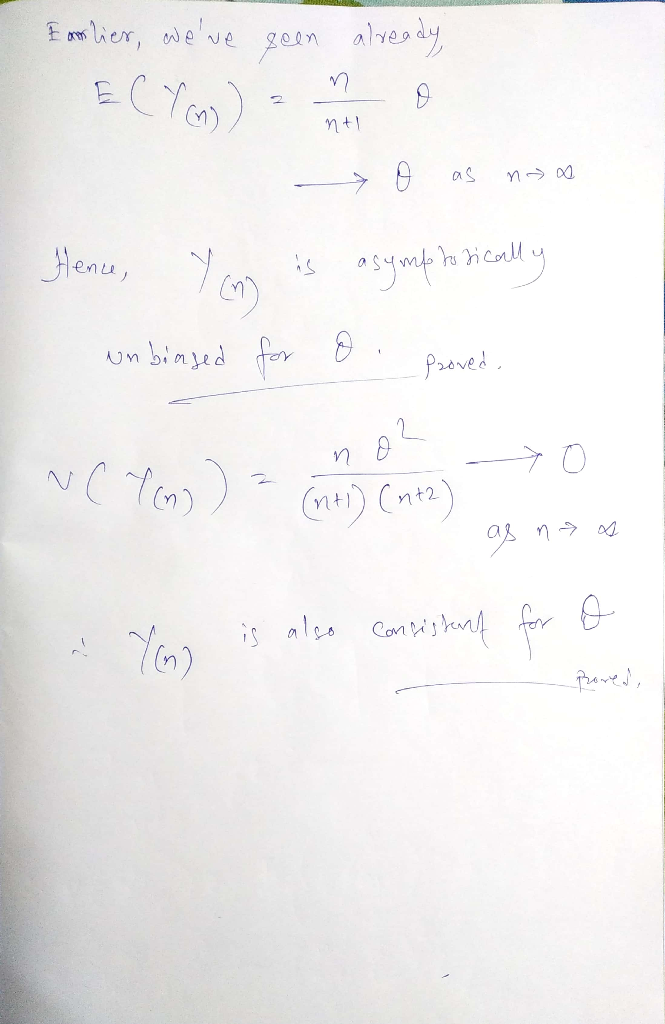##### Add Answer of: 6. In Problem 1, show that θ2 is a consistent estimator for θ. Deduce that Y(n) is a consistent e...
More Homework Help Questions Additional questions in this topic.

• #### consistent estimator proof!

Need Online Homework Help?